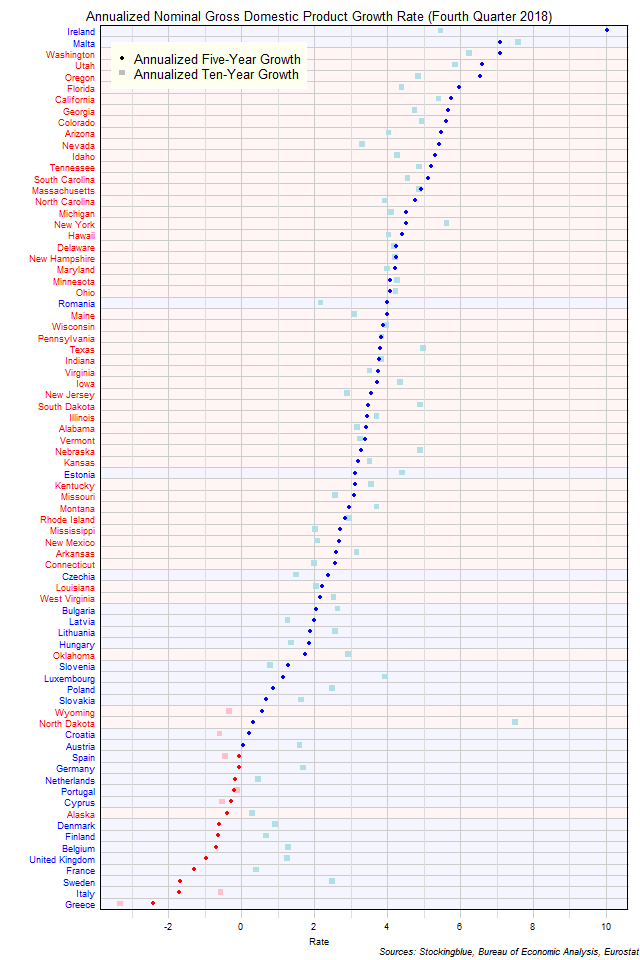# Long-Term EU and US GDP Growth Rate by State, Fourth Quarter 2018

Aug 1, 2019The chart above shows the annualized nominal gross domestic product (GDP) growth rate when the GDP is priced in US dollars in each EU and US state over the past five years as of the fourth quarter of 2018 and the growth rate over the past ten years.  Only EU states saw a contraction both over the past five and ten years.

## Findings

• The difference between the state with the largest annualized five-year growth rate, Ireland, and the state with the smallest, Greece, is 12.45 percentage points.
• The difference between the state with the largest annualized ten-year growth rate, Malta, and the state with the smallest, Greece, is 10.91 percentage points.
• The median annualized five-year growth rate in the 28 EU states is 0.14% and the mean 1.00%.  The median annualized ten-year growth rate in the 28 EU states is 1.33% and the mean 1.54%.
• The median annualized five-year growth rate in the 50 US states is 3.81% and the mean 3.87%.  The median annualized ten-year growth rate in the 50 US states is 3.99% and the mean 3.84%.
• The median annualized five-year growth rate in the 78 EU and US states is 3.17% and the mean 2.84%.  The median annualized ten-year growth rate in the 78 EU and US states is 3.28% and the mean 3.01%.
• Forty states' economies grew faster over the past five years than they did over the past ten years (10 from the EU, 30 from the US).  The remaining 38 states saw their economies grow slower over the past five years than they did over the past ten years (18 EU, 20 US).
• All EU drops in GDP growth rate with the exception of Greece's ten-year drop are attributed to currency rate fluctuations.

## Caveats

• Data is from the fourth quarters of 2018, 2013, and 2018.
• The data is seasonally adjusted in current dollars.
• Euros are converted to dollars at an average exchange rate of 1.14 for the fourth quarter of 2018, 1.36 for the fourth quarter of 2013, and 1.32 for the fourth quarter of 2008 according to historic rates listed at the Federal Reserve (see source link below).
• US data comes in an annualized format which the EU does not, thus EU data is annualized by multiplying the quarterly figure by four.
• US growth rates may differ from those provided by the Bureau of Economic Analysis as the BEA's growth rates are based on chained dollars in conjunction with the chain index or the quality index for real GDP.  The growth rates listed here are based on nominal GDP.
• All figures are rounded to the nearest hundredth.

## Details

Greece had the largest decline over the past five years with an annualized growth rate of of -2.41%.  Ireland had the largest growth with an annualized gain of 10.04%.

Over the past ten years, Greece had the largest decline with an annualized growth rate of -3.33% drop in GDP while Malta had the largest growth with an annualized growth rate of 7.58%.

North Dakota saw the largest decrease in its growth rate between its annualized ten-year growth and its annualized five-year growth slowing its rate by 7.19 percentage points.  Ireland had the largest increase in its growth rate between its annualized ten-year growth and its annualized five-year growth ramping up its rate by 4.58 percentage points.

Nevada and Romania both went up 28 spots in the growth rank: Nevada went from having the 39th largest growth rate over the past ten years to the 11th largest growth rate over the past five years and Romania went from 53rd largest growth to 25th largest growth over the same period.  Conversely, North Dakota went from having the 2nd highest growth rate over the past ten years to the 62nd highest growth rate over the past five years.  Only three states had annualized growth rates in the top five both over the past ten years and over the past five years: Malta (highest annualized growth over ten years, 2nd highest annualized growth over five years), Washington (3rd highest ten-year, 2nd highest five-year), and Utah (4th highest ten-year, 4th highest five-year).  Two states had growth rates in the bottom five both over both periods: Italy (76th highest ten-year, 77th highest five-year) and Greece (78th highest ten-year, 78th highest five-year).

## Sources

Federal Reserve.  2019.  "Foreign Exchange Rates."  Accessed May 26, 2019.  https://www.federalreserve.gov/releases/g5/.

US Bureau of Economic Analysis.  2019.  "GDP by State."  Accessed May 22, 2019.  https://www.bea.gov/data/gdp/gdp-state.